﻿

### Asymptotic behavior for a nonlocal diffusion problem with Neumann boundary conditions and a reaction term

#### Abstract

In this paper, we consider the following initial value problem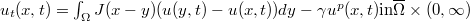$u_t(x,t)=\int_{\Omega}J(x-y)(u(y,t)-u(x,t))dy-\gamma u^{p}(x,t)& \mbox{in}& \overline{\Omega}\times(0,\infty)$,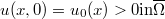$u(x,0)=u_{0}(x)>0& \mbox{in}& \overline{\Omega}$,            where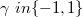$\gamma\ in \{-1,1\}$ is a parameter,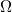$\Omega$ is a bounded domain in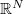$\mathbb{R}^{N}$ with smooth boundary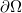$\partial\Omega$,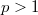$p>1$,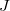$J$: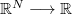$\mathbb{R}^N\longrightarrow\mathbb{R}$ is a kernel which is nonnegative,      measurable, symmetric, bounded and$\int_{\mathbb{R}^N}J(z)dz=1,$ the      initial datum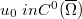$u_0 \ in C^0(\overline{\Omega})$,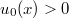$u_0(x)>0$ in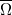$\overline{\Omega}$. We show that, if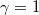$\gamma=1$, then the solution$u$      of the above problem tends to zero as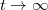$t\rightarrow\infty$ uniformly in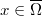$x\in\overline{\Omega}$, and a description of its asymptotic behavior is      given. We also prove that, if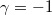$\gamma=-1$, then the solution$u$ blows up      in a finite time, and its blow-up time goes to that of the solution of a      certain ODE as the$L^{\infty}$ norm of the initial datum goes to      infinity.

DOI Code: 10.1285/i15900932v30n1p1

Keywords:
Nonlocal diffusion; asymptotic behavior; blow-up time

Classification: 35B40; 45A07; 35G10

Full Text: PDF